2119

Physics Electromagnetic Induction Level: High School

The 10cm-wide ,zero-resistance slide wire shown in the figure which is shown at ‘click here’ is pushed toward the 2.0 ohm resistor at a steady speed of 0.50 m/s .The magnetic field strength is 0.50 T .

a) How big is the pushing force ?

b) How much power does the pushing force supply to the wire ?

c) What are the direction and magnitude of the induced current ?

d) How much power is dissipated in the resistor ?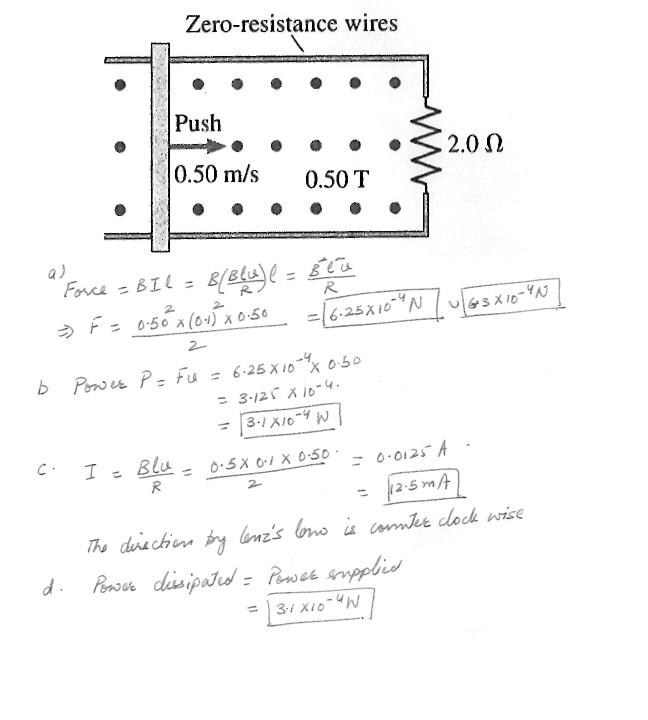2116

Physics Electromagnetic Induction Level: High School

A square wire loop with 2-3m sides is perpendicular to a uniform magnetic field . with half the area of the loop in the field . The loop contain a 2.0 V battery with negligible internal resistance . If the magnitude of the field varies with time according to B = (0.042T)-(0.87T/s)t, what is the total emf of the circuit ?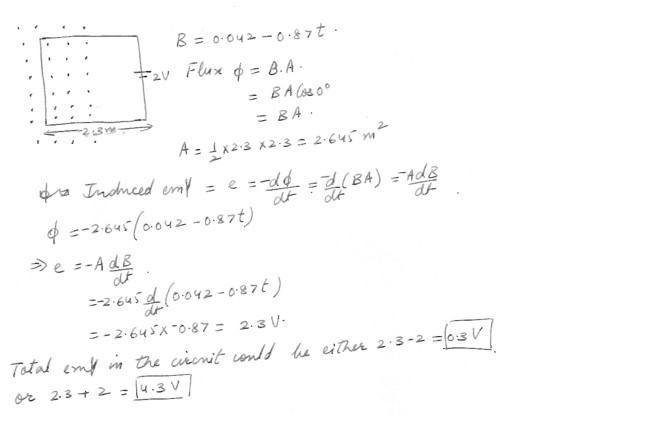2038

Physics Electromagnetic Induction Level: High School

Step up transformer

A transformer charges 120 V across the primary to 1200 V across the secondary . If the secondary coil has 800 turns, how many turns does the primary coil have ?

a) 40

b) 80

c) 100

d) 400

e) 4000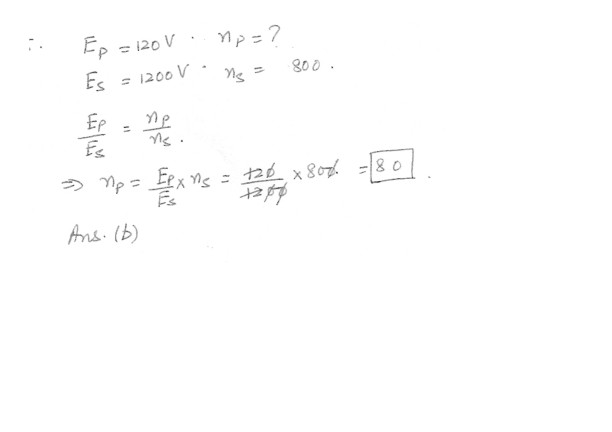2037

Physics Electromagnetic Induction Level: High School

Determine the energy stored in a 95-mH inductor that carries a 1.4-A current.

a) 0.38 J

b) 0.27 J

c) 0.19 J

d) 0.093 J

e) 0.066 J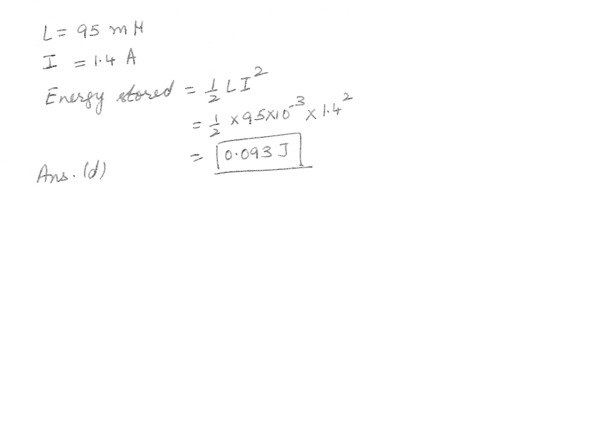2036

Physics Electromagnetic Induction Level: High School

The angular speed of a motor is 262 rad/s. The back emf generated by the motor is 89.4 V. Assuming all other factors remain the same, determine the back emf if the angular speed of the motor is reduced to 154 rad/s .

a) 32.3 V

b) 44.7 V

c) 52.5 V

d) 89.4 V

e) 152 V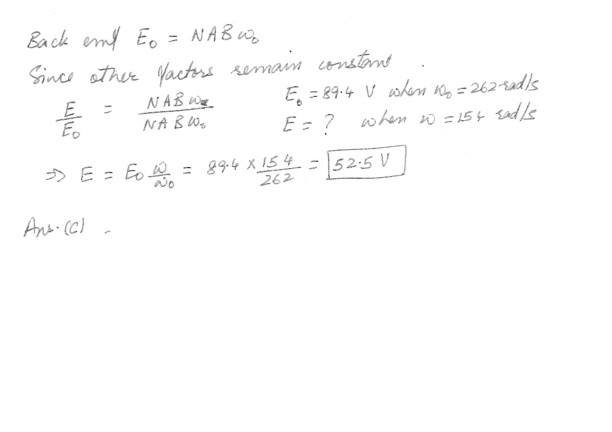2035

Physics Electromagnetic Induction Level: High School

A long straight wire in the same plane as a rectangular , conducting loop . The wire carries a constant current 1 as shown in the figure at “click here” . Which one of the following statements is true if the wire is suddenly moved toward the loop ?

a) There will be no induced emf and no induced current.

b) There will be an induced emf, but no induced current.

c) There will be an induced current that is clockwise around the loop .

d) There will be an induced current that is counterclockwise around the loop.

e) There will be an induced electric field that is clock wise around the loop.2033

Physics Electromagnetic Induction Level: High School

A conducting loop of wire is placed in a magnetic field that is normal to the plane of the loop . Which one of the following actions will not result in an induced current in the loop ?

a) Rotate the loop about an axis that is parallel to the field and passes through the center of the loop .

b) Increase the strength of the magnetic field

c) Decrease the area of the loop

d) Decrease the strength of the magnetic field

e) Rotate the loop about an axis that is perpendicular to the field and passes through the center of the loop .

a) Rotate the loop about an axis that is parallel to the field and passes through the center of the loop .(Because in this case , the flux cutting the loop will not change .)

1954

Physics Electromagnetic Induction Level: High School

Generator – Max emf of 170 V , with angular speed of 3600 rpm . Each coil has area of 0.016 m2 . Magnetic field has magnitude of 0.0050 T , how many turns of wire are needed ?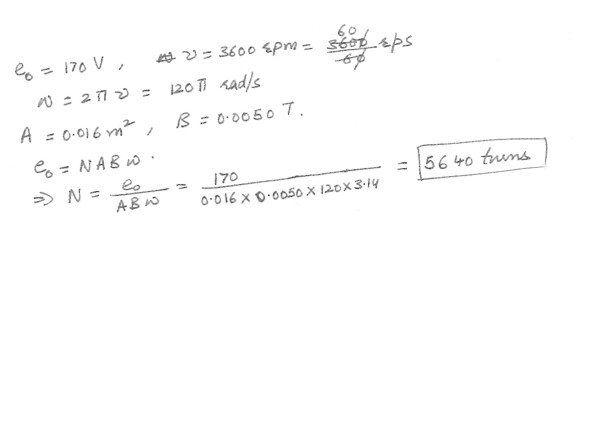1953

Physics Electromagnetic Induction Level: High School

A zero resistance rod sliding to the right on two zero –resistance rails . Rails connected by 12.5 ohm resistor . Magnetic field magnitude = 0.750 T. Find the speed of bar moving with current of 0.125 A in the resistor .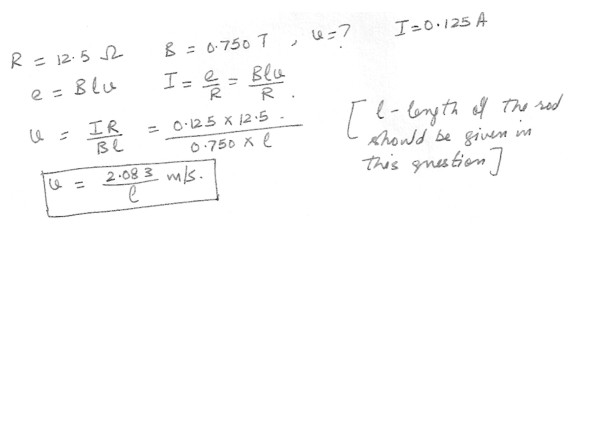1952

Physics Electromagnetic Induction Level: High School

A single conducting loop of wire has an area of 7.4 x 10-2m^2 and a resistance of 100 ohms . Perpendicular to the plan of the loop is a magnetic field of strength 0.18 T . At what rate in (T/s) must this field change if the induced current in the loop is to be 0.22 A ?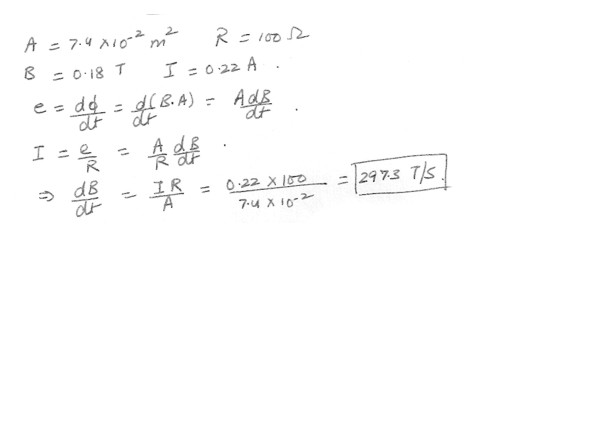Displaying 61-70 of 70 results.